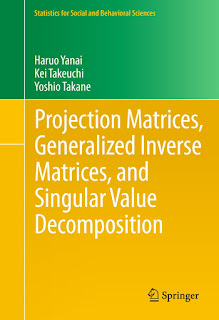MATRIX MATHEMATICS

# Projection Matrices, Generalized Inverse Matrices, and Singular Value Decomposition Free DownloadAside from distribution theory, projections and the singular value decomposition (SVD) are the two most important concepts for understanding the basic mechanism of multivariate analysis. The former underlies the least squares estimation in regression analysis, which is essentially a projection of one subspace onto another, and the latter underlies principal component analysis, which seeks to find a subspace that captures the largest variability in the original space.This book is about projections and SVD. A thorough discussion of generalized inverse (g-inverse) matrices is also given because it is closely related to the former. The book provides systematic and in-depth accounts of these concepts from a unified viewpoint of linear transformations finite dimensional vector spaces. More specially, it shows that projection matrices (projectors) and g-inverse matrices can be defined in various ways so that a vector space is decomposed into a direct-sum of (disjoint) subs paces. Projection Matrices, Generalized Inverse Matrices, and Singular Value Decomposition will be useful for researchers, practitioners, and students in applied mathematics, statistics, engineering, behavior metrics, and other fields.